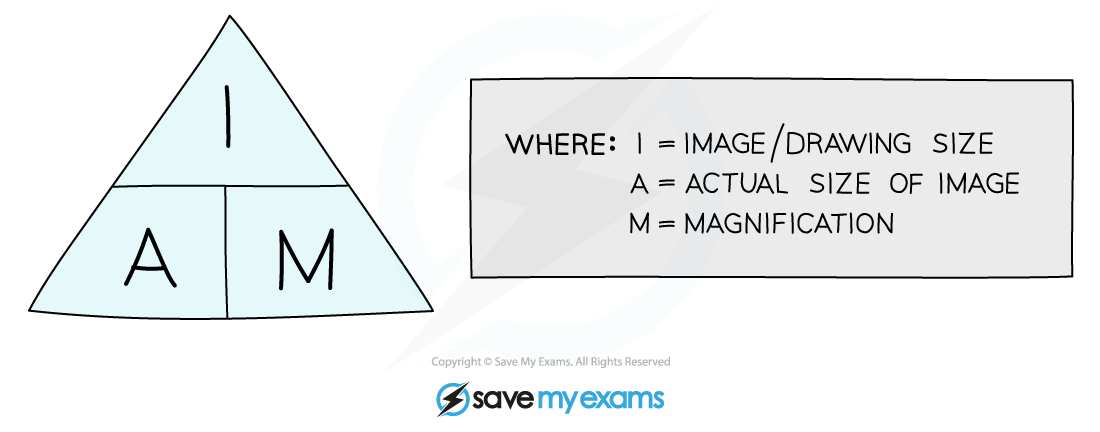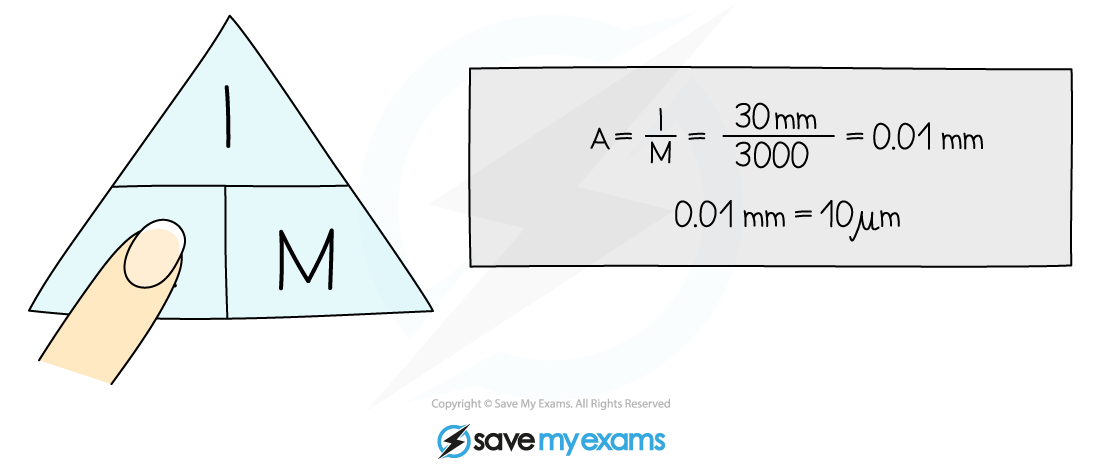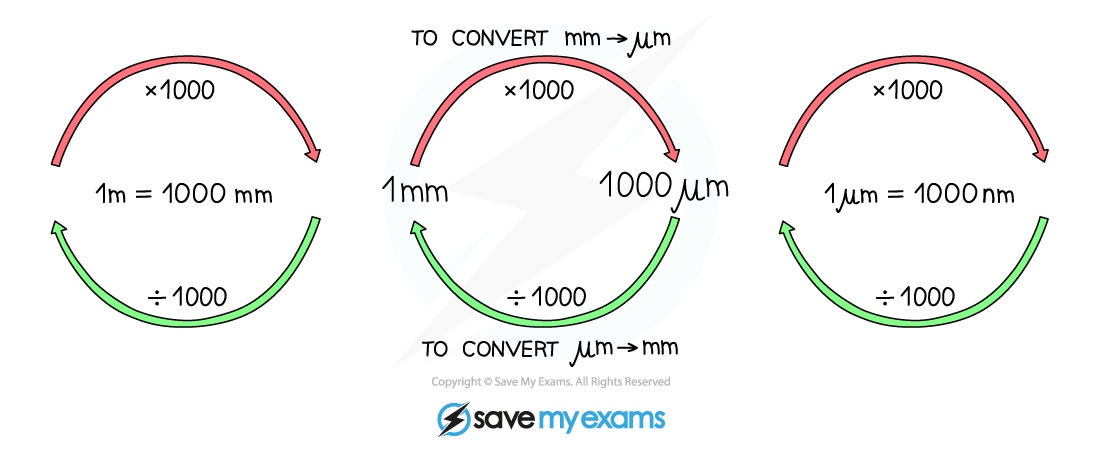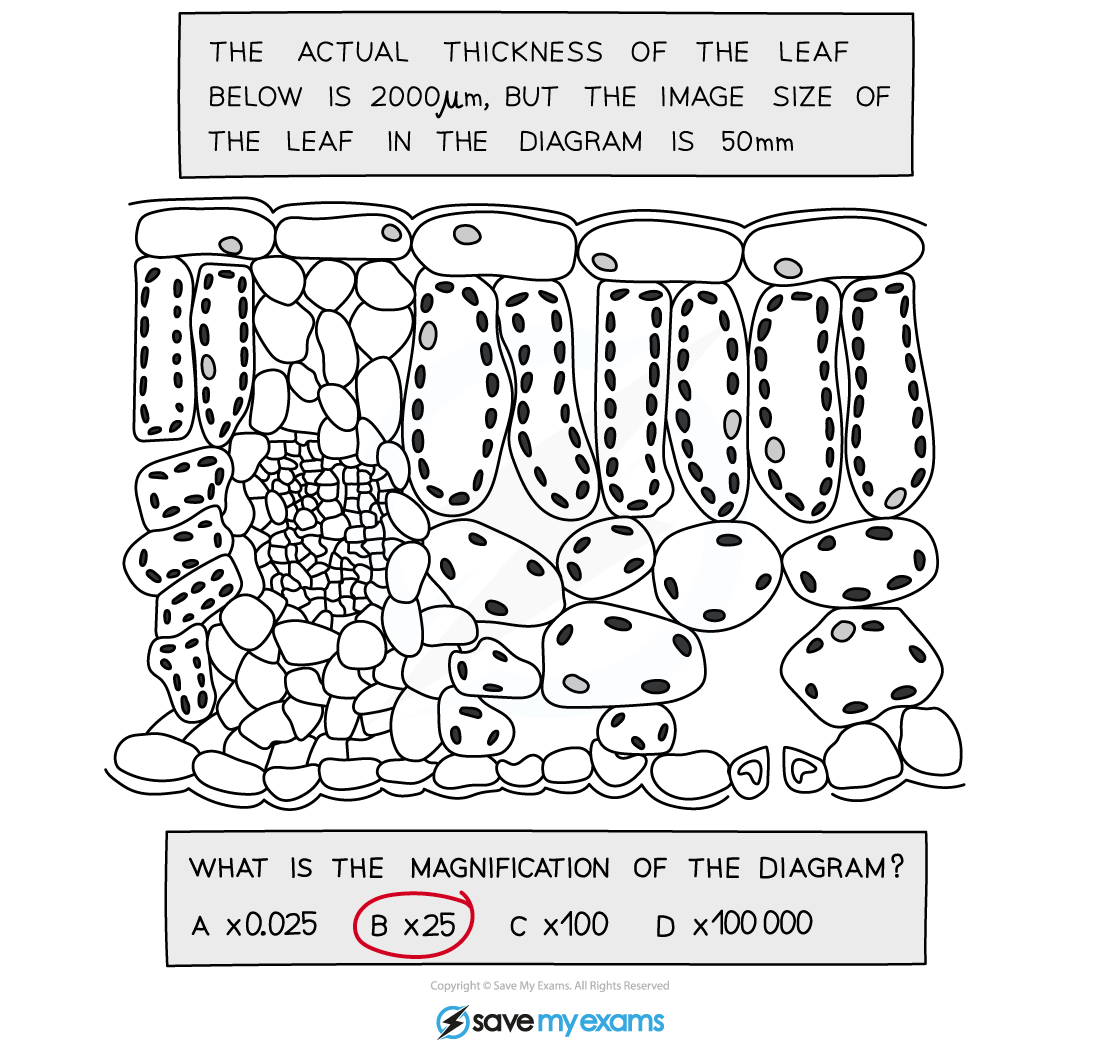# AQA A Level Biology复习笔记2.2.5 Magnification Calculations

### Magnification Calculations

• Magnification is how many times bigger the image of a specimen observed is in comparison to the actual (real-life) size of the specimen
• The magnification (M) of an object can be calculated if both the size of the image (I), and the actual size of the specimen (A), is knownAn equation triangle for calculating magnification

#### Worked Example

An image of an animal cell is 30 mm in size and it has been magnified by a factor of X 3000.

What is the actual size of the cell?

To find the actual size of the cell:• The size of cells is typically measured using the micrometre (μm) scale, with cellular structures measured in either micrometers (μm) or nanometers (nm)
• When doing calculations all measurements must be in the same units. It is best to use the smallest unit of measurement shown in the question
• To convert units, multiply or divide depending if the units are increasing or decreasing
• Magnification does not have units

Converting units of measurement• There are 1000 nanometers (nm) in a micrometre (µm)
• There are 1000 micrometres (µm) in a millimetre (mm)
• There are 1000 millimetres (mm) in a metre (m)

#### Worked ExampleStep 1: Check that units in magnification questions are the same

Remember that 1mm = 1000µm

2000 / 1000 = 2, so the actual thickness of the leaf is 2 mm and the drawing thickness is 50 mm

Step 2: Calculate Magnification

Magnification = image size / actual size = 50 / 2 = 25

So the magnification is x 25